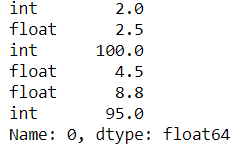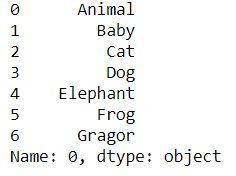# Pandas.DataFrame.iterrows() function in Python

• Difficulty Level : Basic
• Last Updated : 23 May, 2022

Pandas DataFrame.iterrows() is used to iterate over a pandas Data frame rows in the form of (index, series) pair. This function iterates over the data frame column, it will return a tuple with the column name and content in form of series.

Syntax: DataFrame.iterrows() Yields: index- The index of the row. A tuple for a MultiIndex data- The data of the row as a Series Returns: it: A generator that iterates over the rows of the frame

Example 1:

Sometimes we need to iter over the data frame rows and columns without using any loops, in this situation Pandas DataFrame.iterrows() plays a crucial role.

## Python3

 `import` `pandas as pd`   `# Creating a data frame along with column name` `df ``=` `pd.DataFrame([[``2``, ``2.5``, ``100``, ``4.5``, ``8.8``, ``95``]], columns``=``[` `                  ``'int'``, ``'float'``, ``'int'``, ``'float'``, ``'float'``, ``'int'``])`   `# Iter over the data frame rows` `# # using df.iterrows()` `itr ``=` `next``(df.iterrows())[``1``]` `itr`

Output:In the above example, we use Pandas DataFrame.iterrows() to iter over numeric data frame rows.

Example 2:

## Python3

 `import` `pandas as pd`   `# Creating a data frame` `df ``=` `pd.DataFrame([[``'Animal'``, ``'Baby'``, ``'Cat'``, ``'Dog'``,` `                    ``'Elephant'``, ``'Frog'``, ``'Gragor'``]])`   `# Iterating over the data frame rows` `# using df.iterrows()` `itr ``=` `next``(df.iterrows())[``1``]` `itr`

Output :In the above example, we iter over the data frame having no column names using Pandas DataFrame.iterrows()

Note: As iterrows returns a Series for each row, it does not preserve dtypes across the rows.

My Personal Notes arrow_drop_up
Recommended Articles
Page :